Homework Help Question & Answers

# Find two power series solutions of the given differential equation about the ordinary point x =...

Find two power series solutions of the given differential equation about the ordinary point x = 0. y′′ − 4xy′ + y = 0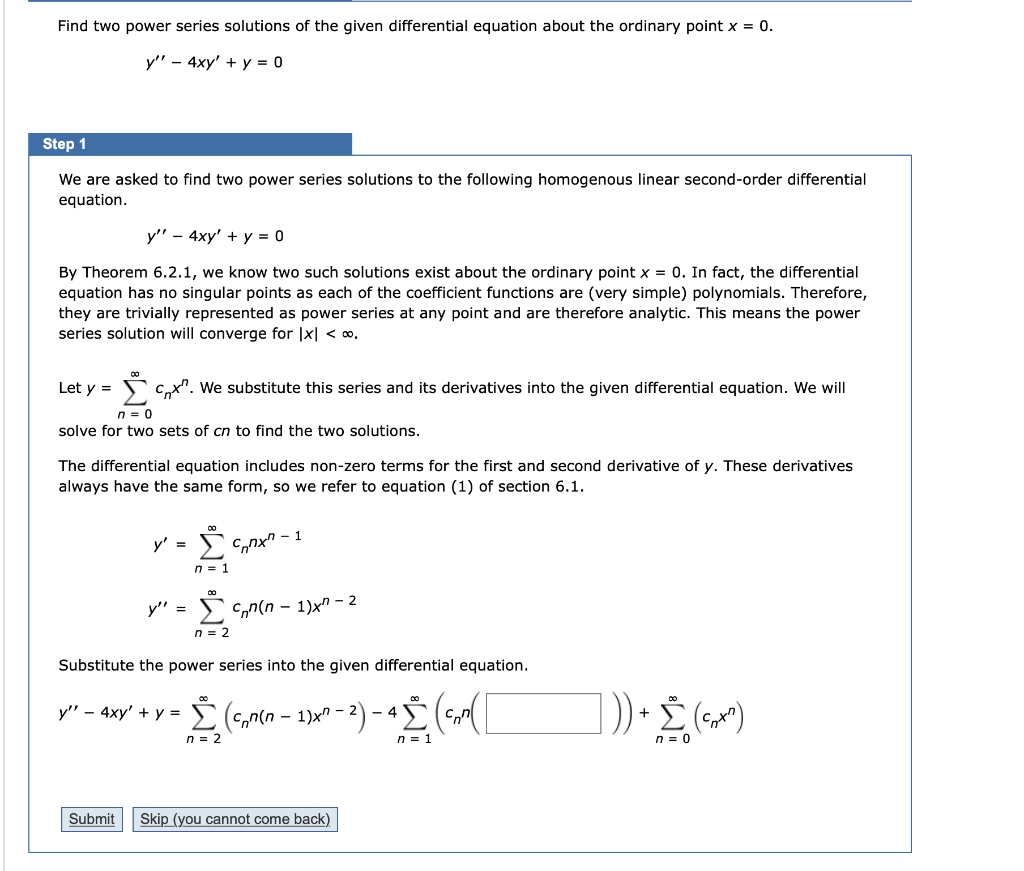Find two power series solutions of the given differential equation about the ordinary point x = 0. y!' - 4xy' + y = 0 Step 1 We are asked to find two power series solutions to the following homogenous linear second-order differential equation. y" - 4xy' + y = 0 By Theorem 6.2.1, we know two such solutions exist about the ordinary point x = 0. In fact, the differential equation has no singular points as each of the coefficient functions are very simple) polynomials. Therefore, they are trivially represented as power series at any point and are therefore analytic. This means the power series solution will converge for 1x1 < 0. Let y = n = 0 solve for two sets of cn to find the two solutions. to cox". We substitute this series and its derivatives into the given differential equation. We will The differential equation includes non-zero terms for the first and second derivative of y. These derivatives always have the same form, so we refer to equation (1) of section 6.1. y' = ☺ canxa - 1 n = 1 y" = n(n - 1)x7 - 2 n = 2 Substitute the power series into the given differential equation. y" - 4xy' + y = Š (c, n(n − 1)x9 2) - 4 (C (CMX") n = 2 n=1 n = 0 Submit Skip (you cannot come back)

#### Homework Answers

Answer #1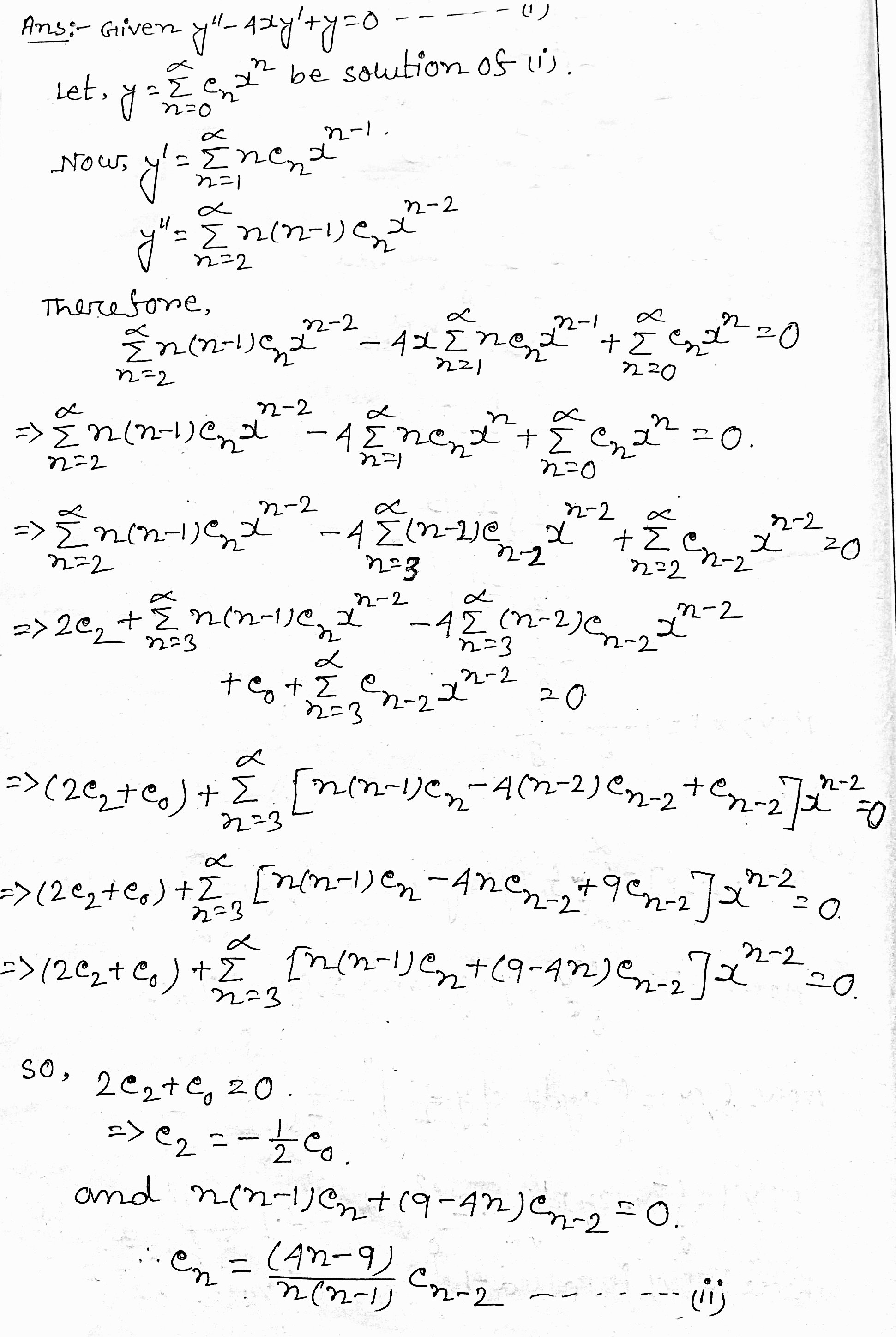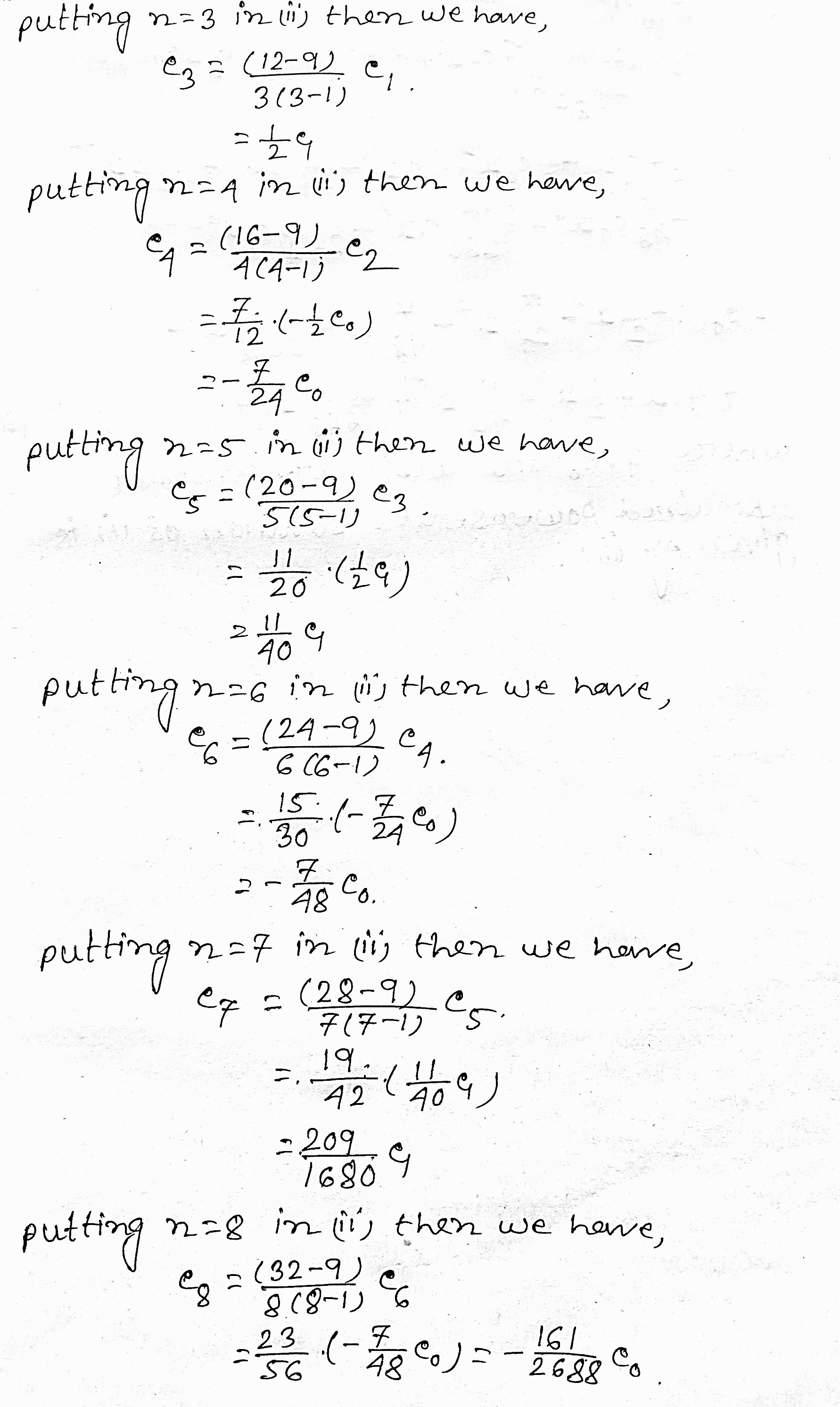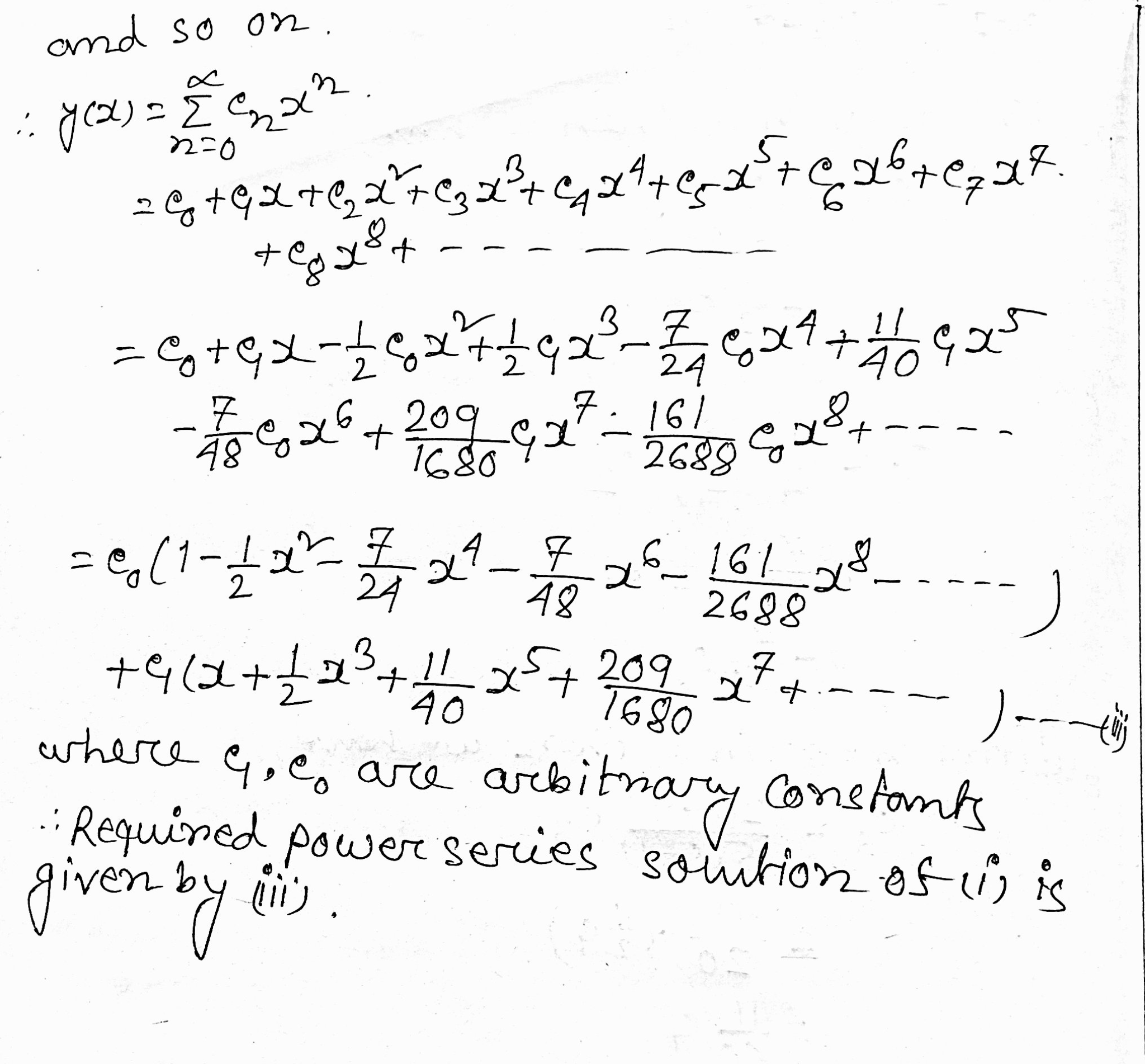Know the answer?
Your Answer:

#### Post as a guest

Your Name:

What's your source?

#### Earn Coin

Coins can be redeemed for fabulous gifts.

Not the answer you're looking for? Ask your own homework help question. Our experts will answer your question WITHIN MINUTES for Free.
Similar Homework Help Questions
• ### Find two power series solutions of the given differential equation about the ordinary point x =...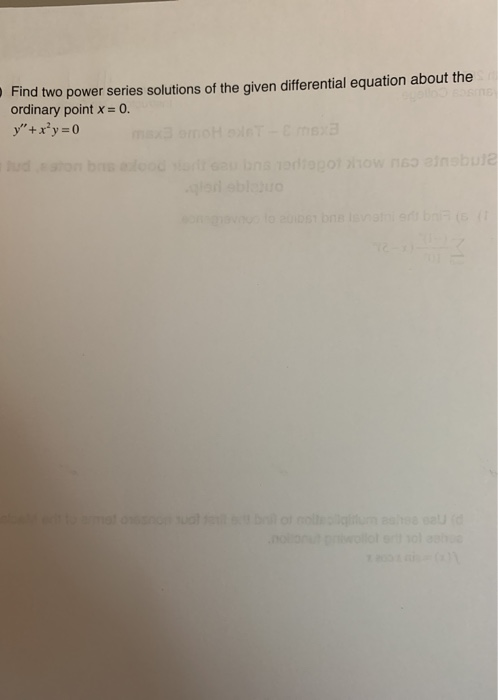Find two power series solutions of the given differential equation about the ordinary point x = 0. y"+x²y=0 dan be n e od polow 165 inebuia VODSTONE l ol lol lol ooroolol

• ### Find two power series solutions of the given differential equation about the ordinary point x=0. (x^2+2)y"+6xy'-y=0...

Find two power series solutions of the given differential equation about the ordinary point x=0. (x^2+2)y"+6xy'-y=0 (Show all steps using y= please) nfiniti

• ### 1) Find two power series solutions of the differential equation (x² + 1)y" – xy' +...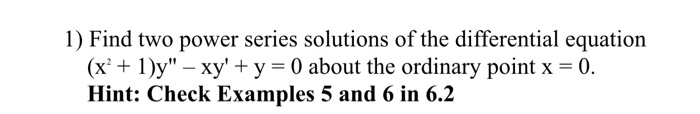1) Find two power series solutions of the differential equation (x² + 1)y" – xy' + y = 0 about the ordinary point x = 0. Hint: Check Examples 5 and 6 in 6.2 Example 6 Power Series Solution Solve (x + 1)," + xy - y = 0. Solution As we have already seen the given differential equation has singular points at = = ti, and so a power series solution centered at o will converge at least for...

• ### Find a recurrence relation for the power series solutions of differential equation y" - 2xy' +...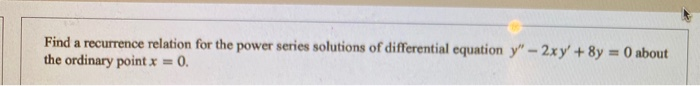Find a recurrence relation for the power series solutions of differential equation y" - 2xy' + 8y = 0 about the ordinary point x = 0.

• ### differential equations 1 +.. 8 Find two power series solutions of the given differential equation about...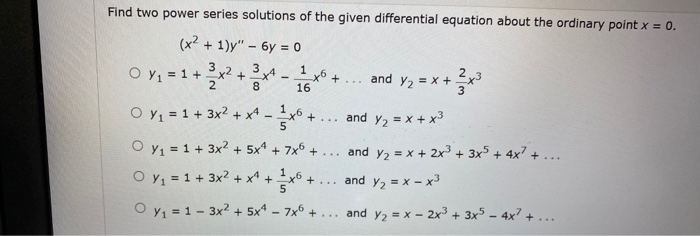differential equations 1 +.. 8 Find two power series solutions of the given differential equation about the ordinary point x = 0. (x2 + 1)" - 6y = 0 O Y1 = 1 + x2 + 3x4 xo and Y2 = x = x + 3x3 16 O x1 = 1 + 3x2 + x4 – xo + and y2 = x + x3 O Y1 = 1 + 3x2 + 5x* + 7x® + ... and y2 = x...

• ### Use a power series centered about the ordinary point x0 = 0 to solve the differential equation (x − 4)y′′ − y′ + 12xy =...

Use a power series centered about the ordinary point x0 = 0 to solve the differential equation (x − 4)y′′ − y′ + 12xy = 0 Find the recurrence relation and at least the first four nonzero terms of each of the two linearly inde- pendent solutions (unless the series terminates sooner). What is the guaranteed radius of convergence?

• ### The power series solution of the differential equation y" - xy'+y=0 about the ordinary point x...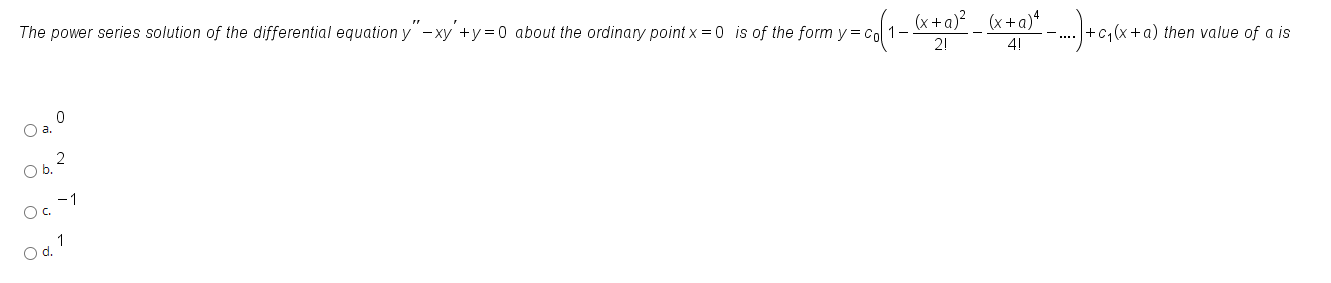The power series solution of the differential equation y" - xy'+y=0 about the ordinary point x =0 is of the form y=col =cod (x+2)? _ (x + a)-...)+cq6x + a) then value of a is 0 O a. 062 Oc -1 O01

• ### 2. Find two power series solutions and give the general solution about the ordinary point i...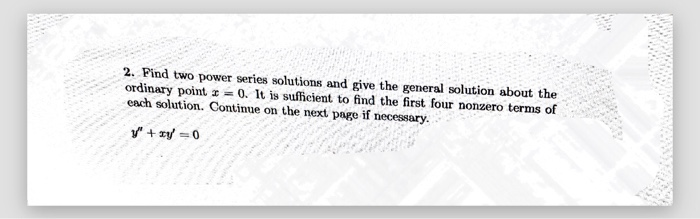2. Find two power series solutions and give the general solution about the ordinary point i = 0. It is suffeient to find the first four nonzero terms of each solution. Continue on the next page if necessary. y" + xy = 0

• ### Seek power series solution of the given differential equation about the given point x0; find the recurrence relation

Seek power series solution of the given differential equation about the given point x0; find the recurrence relation.(1-x)y'' + y = 0; x0 = 0

• ### Solutions about ordinary points For the given DEs , a. Indicate any singular points. b. Find the minimum radius of convergence of the power series solutions about the ordinary point

Solutions about ordinary points For the given DEs , a. Indicate any singular points. b. Find the minimum radius of convergence of the power series solutions about the ordinary point

Free Homework App

Scan Your Homework
to Get Instant Free Answers
Need Online Homework Help?

Get Answers For Free
Most questions answered within 3 hours.